﻿ ProSpeakers Forum - Re: Hornsub shootout RESULTS - 12π - Wayne Parham, October 15, 2005 at 14:20:02# Re: Hornsub shootout RESULTS - 12π

[ ProSpeakers Forum ] [ Help ]

Posted by Wayne Parham [ 67.66.231.14 ] on October 15, 2005 at 14:20:02:

In Reply to: Re: Hornsub shootout RESULTS posted by Wayne Parham on October 15, 2005 at 12:02:43:### 12π basshorn sub

The 12π basshorn sub was next. It weighs in at about 230 lbs and measures 45" x 45" x 28". It uses a pair of LAB12 drivers connected in a push-pull configuration with a heat exchanger for each motor.

Specifications:

Weight: 230 lbs
Dimensions: 45" x 45" x 28"
Power handling: 1600wrms
Impedance: 4Ω
Frequency range: 30Hz - 170Hz
Sensitivity: 105dB/W/M
Max Output: 137dB/M
Distortion at 100 watts: <1%

First, we measured impedance and found Zmin to be 2.7Ω. So 16.5v is required for 100 watts, 23.2v for 200 watts, 32.8v for 400 watts, 46.5v for 800 watts and 65.7v for 1600 watts.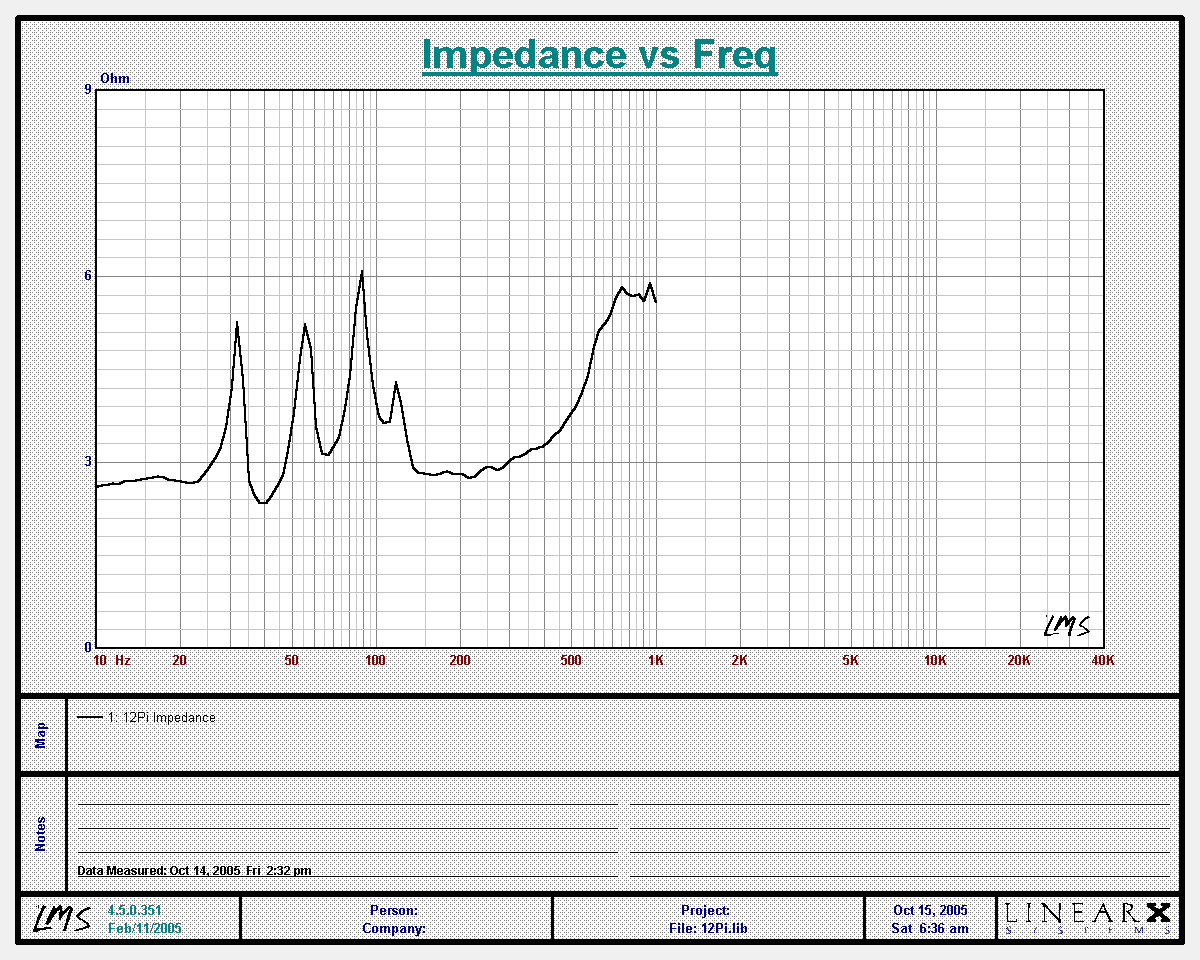### 12π impedance

Next, we measured output at 28.28v. Since we measured 10 meters away, the values correspond to 2.83v/1M.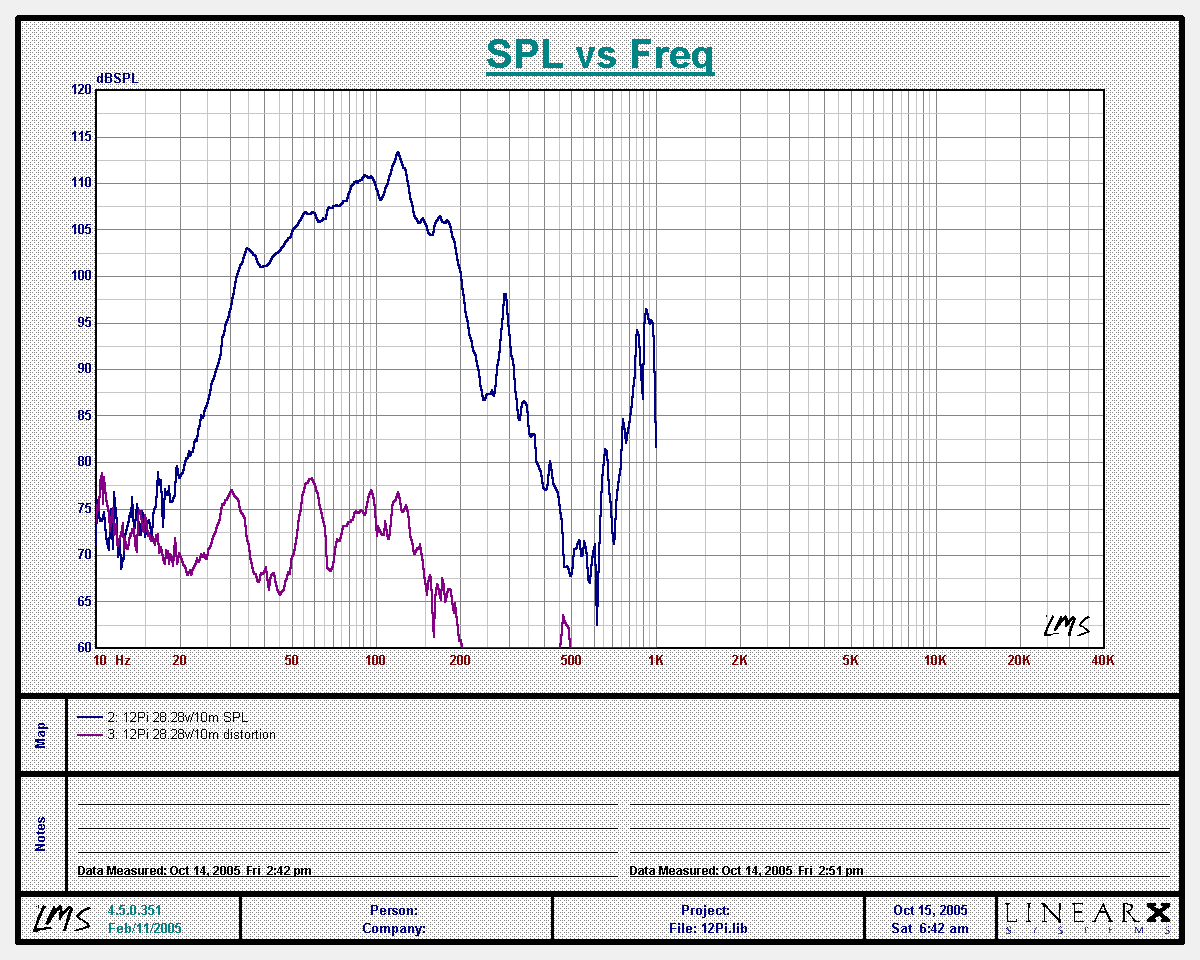### 12π response at 10 meters with 28.28v input (2.83v/1M)

From this, we perform an inverse FFT to obtain impulse and step response: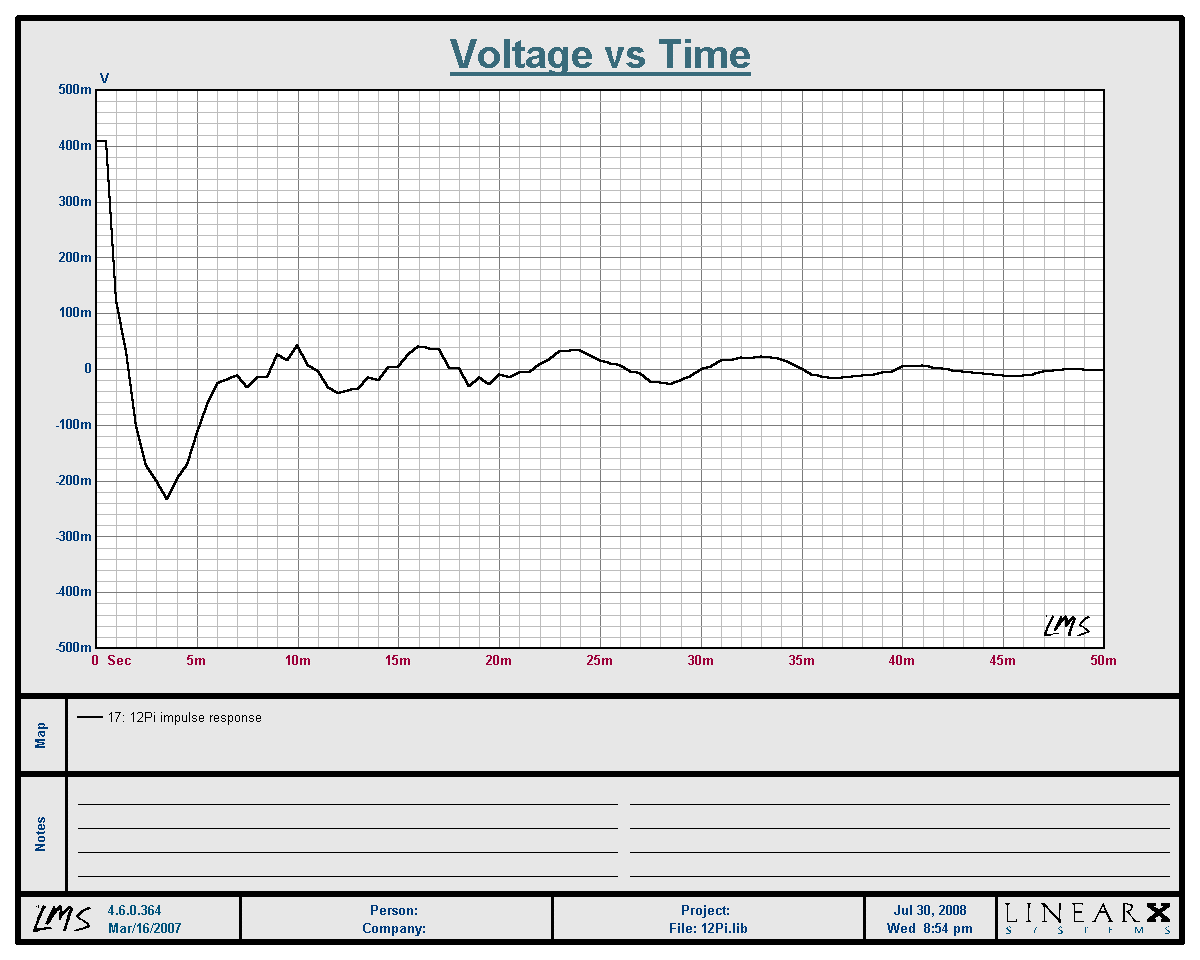### 12π Impulse Response### 12π Step Response

Now to measure output at 100 watts. Since we measured 10 meters away, the values correspond to 1W/1M.

Measurement at 100 watts: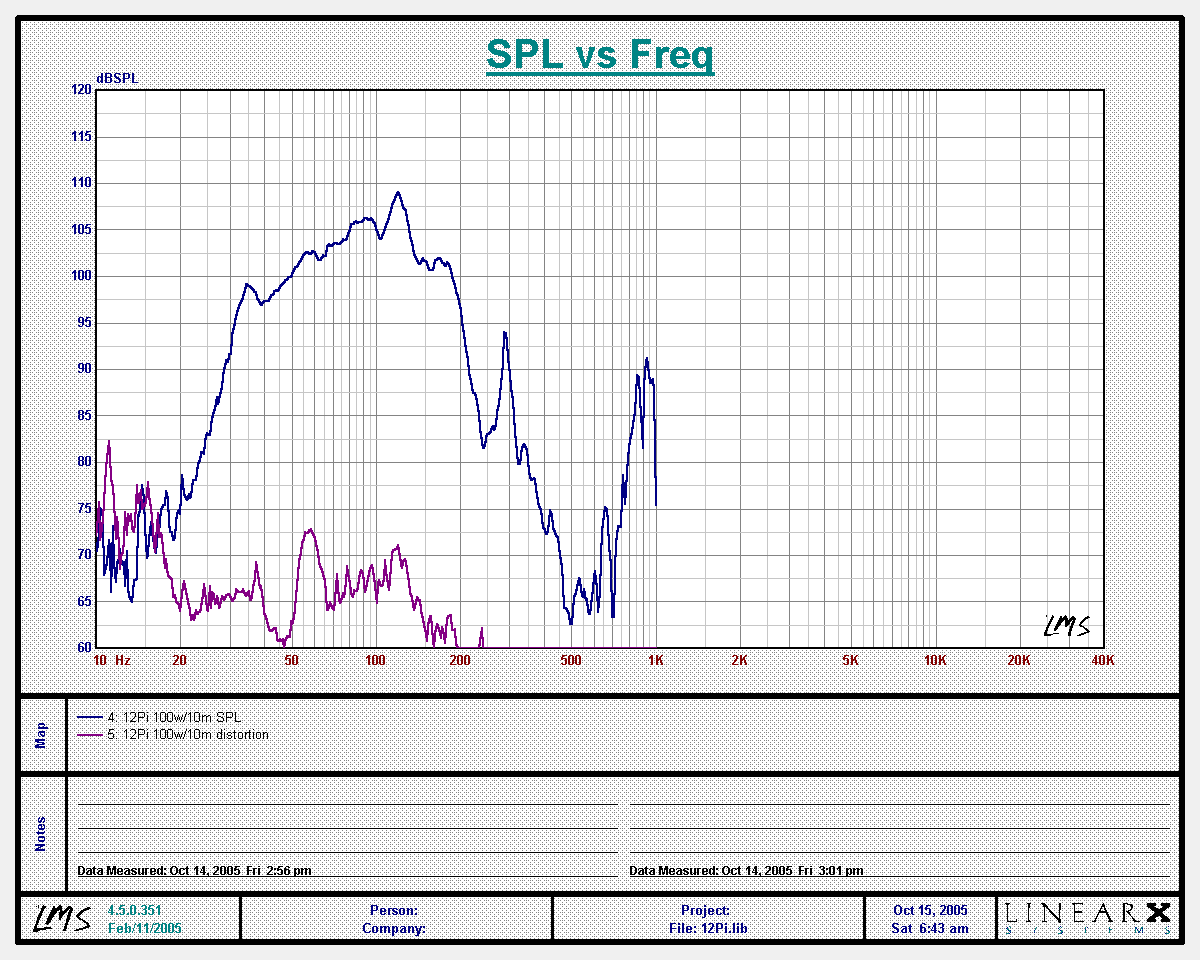### 12π response at 10 meters with 16.5v (100w) input

Output at 100 watts measured at 10 meters is about 106dB average between 60Hz and 160Hz, which is 126db at 1 meter. The blue line is SPL, and the violet line shows distortion. Average distortion is 40dB under the fundamental, which is about 1%.

Measurement at 200 watts: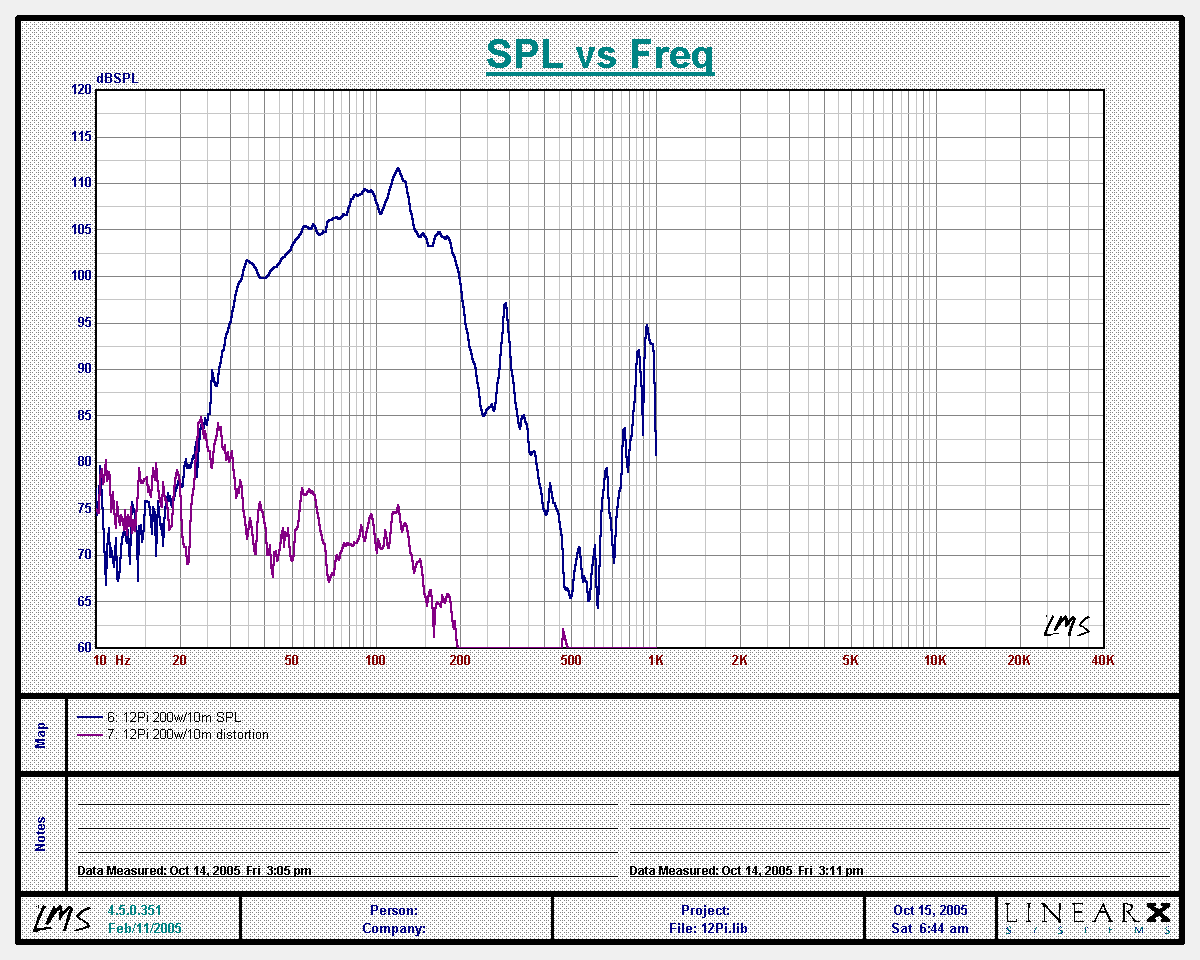### 12π response at 10 meters with 23.2v (200w) input

Output at 200 watts measured at 10 meters is about 109dB average between 60Hz and 160Hz, which is 129db at 1 meter.

Measurement at 400 watts:### 12π response at 10 meters with 32.8v (400w) input

Output at 400 watts measured at 10 meters is about 112dB average between 60Hz and 160Hz, which is 132db at 1 meter.

Measurement at 800 watts: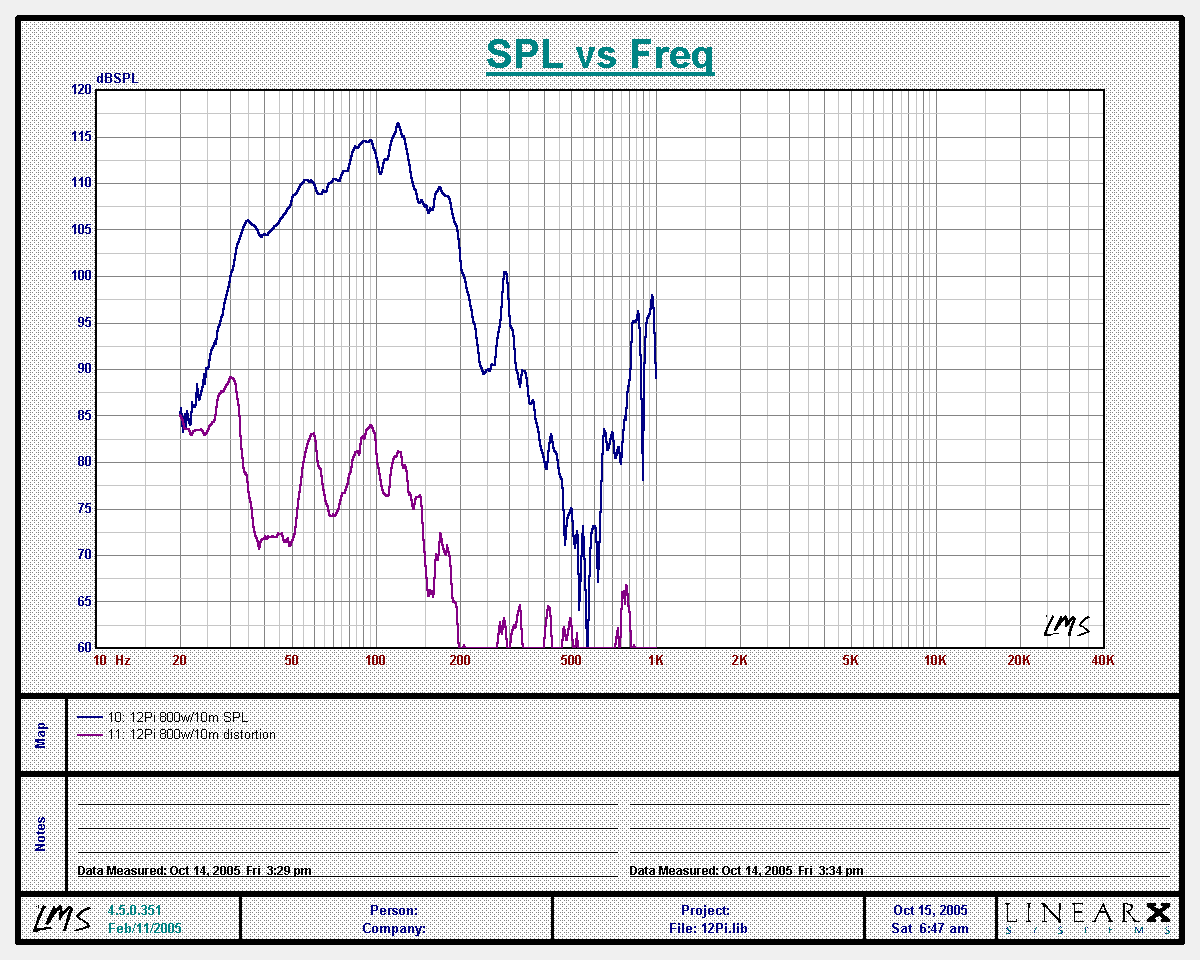### 12π response at 10 meters with 46.5v (800w) input

Output at 800 watts measured at 10 meters is about 115dB average between 60Hz and 160Hz, which is 135db at 1 meter.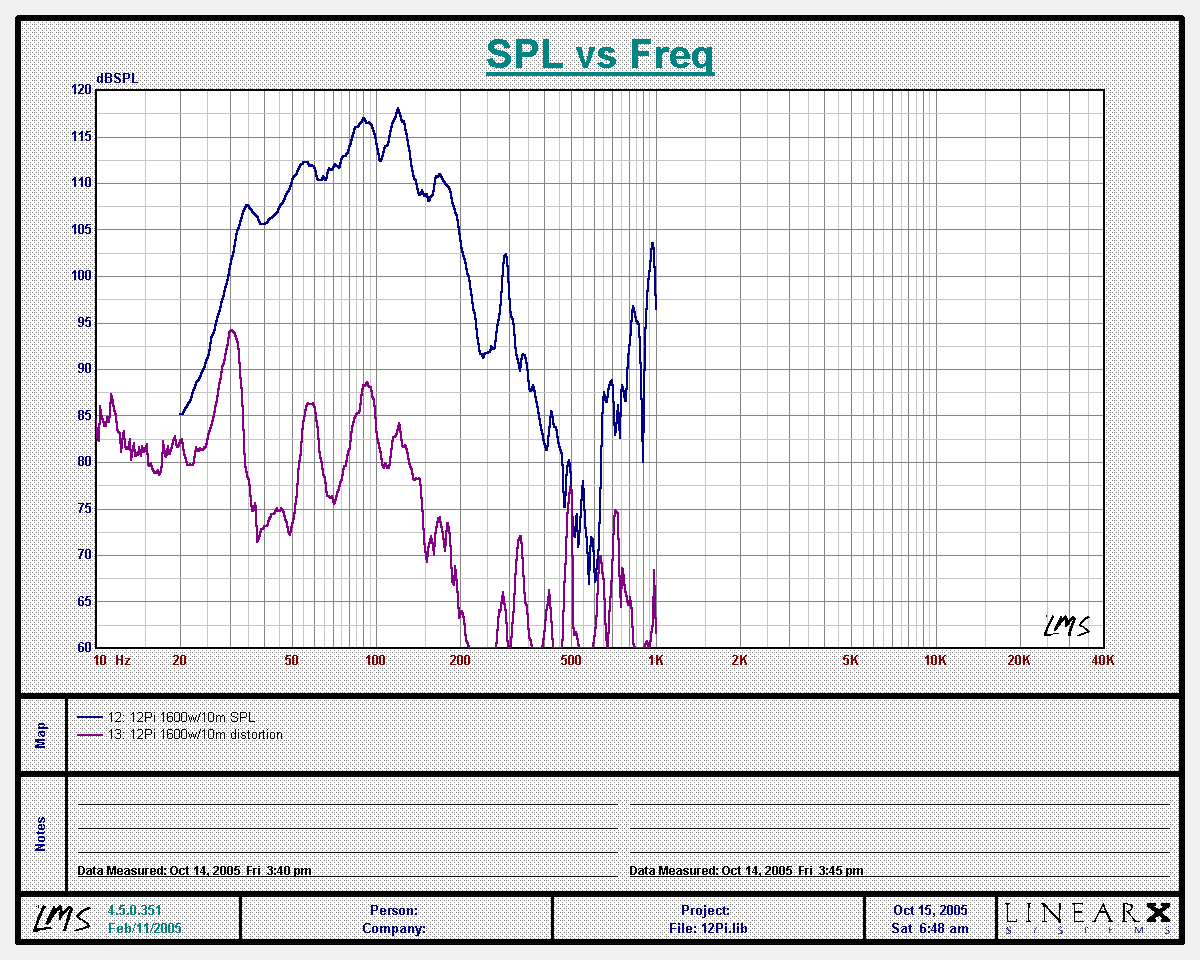### 12π response at 10 meters with 65.7v (1600w) input

Output at 1600 watts measured at 10 meters is about 117dB average between 60Hz and 160Hz, which is 137db at 1 meter.

You can see that compression has started to set in because the increase from 800 watts to 1600 watts was not 3dB, only 2dB. It has a peak at 120Hz, so maximum continuous output is 138dB/M at that frequency. But the point is that there is nothing more to be gained from increasing power above 1600 watts.

Notice that the distortion sweep was run from 10Hz. Even at 1600 watts, mechanical excursion is not excessive at 10Hz. There was no sign of mechanical interference or stress, and the distortion levels are quite low.

This is the limit of the loudspeaker, but I wanted to go further to see how it would act. Specifically, I wanted to see if a mechanical limit was reached before thermal failure. So I ran a test at 2000 watts.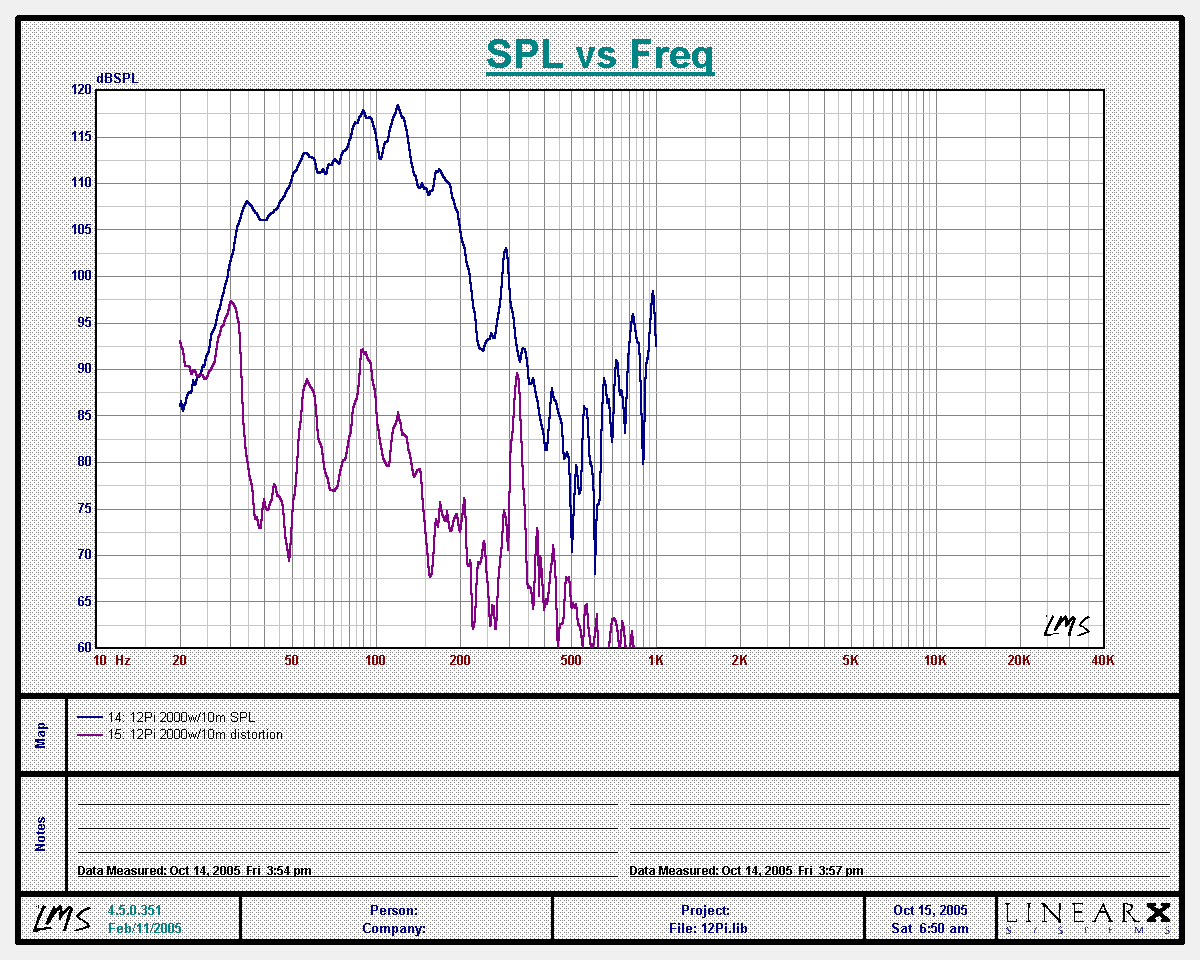### 12π response at 10 meters with 73.5v (2000w) input

Output at 2000 watts measured at 10 meters is about 117dB average between 60Hz and 160Hz, which is 137db at 1 meter. There has been almost no increase in output with the added power. Of course, the increase in power was less than 2dB, but you can see that the increase in sound output was less than that. The power level was well in excess of the speaker's thermal limits but had not exceeded its mechanical limits. It actually sounded powerful and strong, even at this extreme level. Distortion is still quite low. So I tried it at 2400 watts.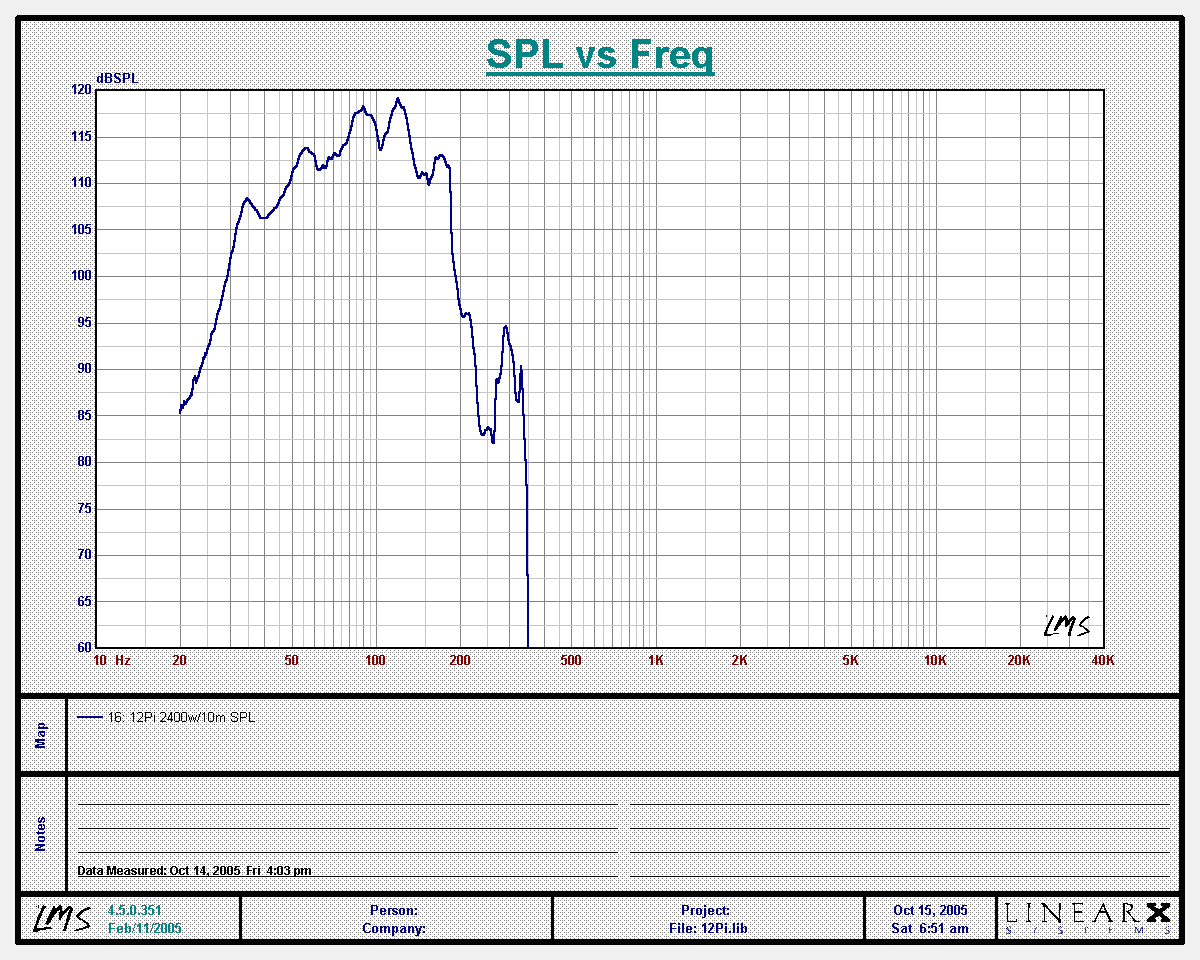### 12π response at 10 meters with 80.5v (2400w) input

Output at 2400 watts measured at 10 meters is about 118dB average between 60Hz and 160Hz, which is 138db at 1 meter. The response curve looks good, but this is so far above the power limits of the driver that the voice coil fused near the end of the sweep. It did not exceed its mechanical limits at any frequency above 20Hz.

[ ProSpeakers Forum ] [ Help ]